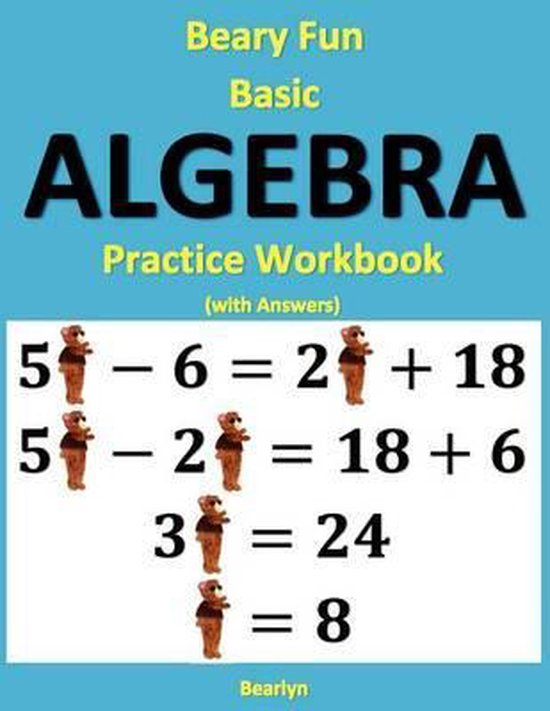# Beary Fun Basic Algebra Practice Workbook (with Answers)

#### Bearlyn

• Bindwijze: Paperback
• Taal: Engels
• Categorie: Onderwijs & Didactiek
• Uitgever: Createspace Independent Publishing Platform
• ISBN: 9781480150089
Inhoud
 Taal: Engels Bindwijze: Paperback Druk: 1 Verschijningsdatum: december 2012 Afmetingen: Afmeting: 27,9 x 21,6 x 0,8 cm Aantal pagina's: 144 pagina's Aanbevolen leeftijd: 6 - 9 jaar Illustraties: Nee
Betrokkenen
 Auteur(s): Bearlyn Auteur(s): Bearlyn

#### Samenvatting

CONCEPT: This algebra workbook has a creative twist: A picture of a Cuddly Bear is used in place of a boring old X for the unknown. The idea behind adding this artistic touch to the equations is to help make algebra seem a little more fun and enjoyable. Students don't need to draw bears in their solutions, though - they can write X if they want. However, there is an opportunity for students to be creative - for example, they could draw smiley faces instead of X's. INSTRUCTION: The staff at Al-Bear Einstein developed a concise, one-page introduction to each chapter that explains what to do in simple terms and provides an instructive example to serve as a guide. The description emphasizes the underlying concept, like dividing both sides by the same number. The material is presented in stages: A new technique is introduced in each stage, allowing students to focus on and master one idea at a time. Review sections help to reinforce the older material and force students to learn when to apply the various techniques. The Cuddly Bears drawn by artist Bearlyn help to engage student interest and make the learning process fun. STRUCTURE: This workbook offers plenty of practice applying basic algebra skills to solve equations. The problems start out simple, like X + 4 = 12, and progressively advance, culminating in equations like 3 X + 8 = 9 X - 16 (but with Cuddly Bears instead of X's). The problems are written in a large font. ANSWERS: Teachers or parents can check the answers at the end of the workbook. LEVEL. This workbook focuses on basic algebra skills. No prior experience with algebra is needed. The goal is to learn how to solve for one unknown in a linear equation using basic arithmetic operations. All of the answers are positive integers. There are no fractions. All of the problems can be solved without a calculator with elementary arithmetic with the numbers 1 thru 9. This way, students can focus on the main idea without distracting complications. CONTENTS: The stages of this workbook are presented as follows: This workbook begins with simple equations that can be solved with a single arithmetic operation, like adding a constant to both sides. Each of the first four sections focuses on a different operation. This allows students to master one technique at a time. A review section mixes the four operations together. The next section combines operations together. For example, it may be necessary to first subtract a number from both sides and then divide both sides by a number. Students next learn how to add the unknown to both sides of the equation in order to solve the problem. The last section offers ample practice with equations of the form 3 X + 8 = 23 - 2 X. Students must add or subtract both constants and unknowns to/from both sides of the equation in order to solve these problems.